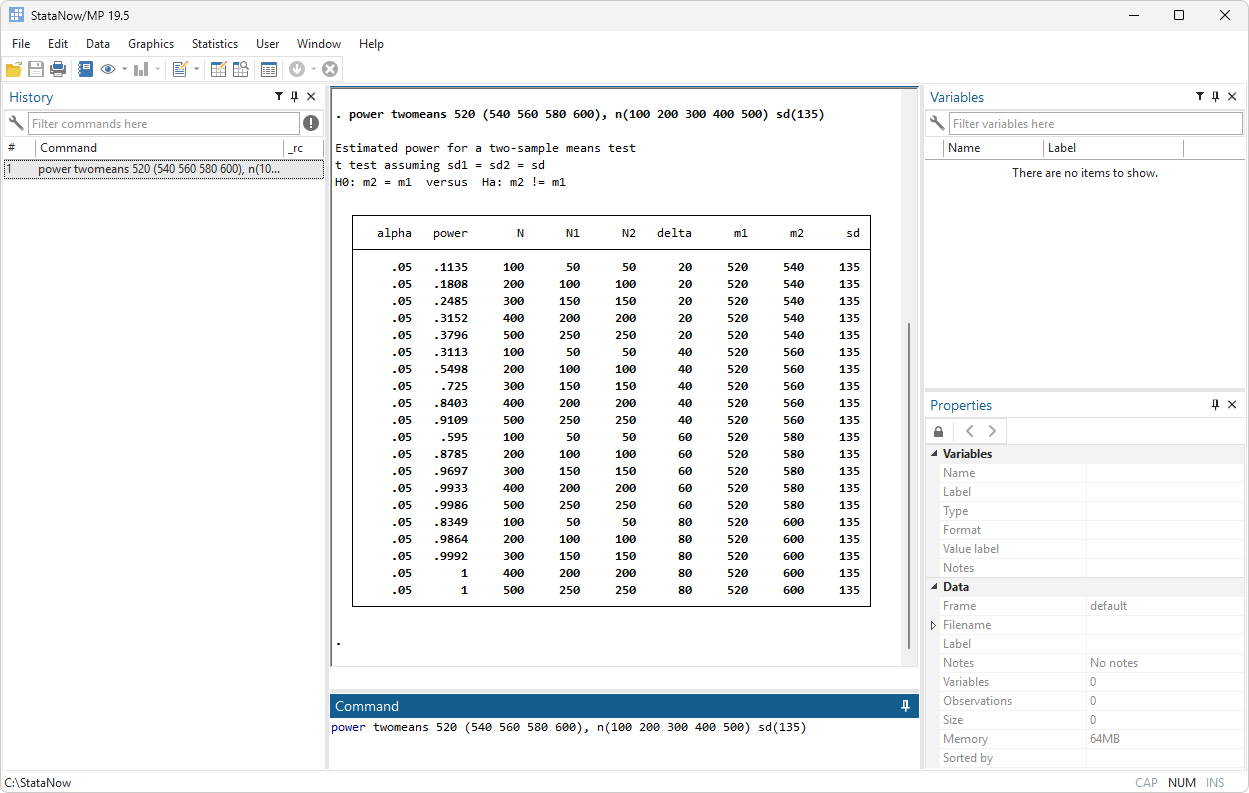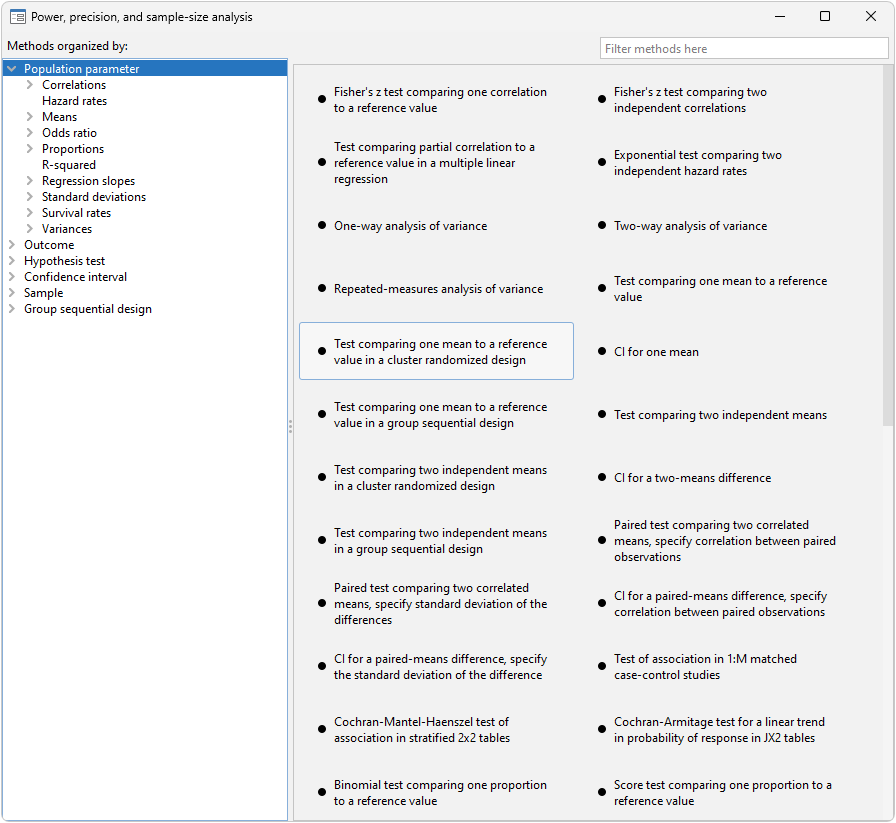Before you conduct your experiment, determine the sample size needed to detect meaningful effects without wasting resources. Do you intend to compute confidence intervals (CIs) or perform hypothesis tests? For hypothesis testing, use Stata’s power commands or interactive Control Panel to compute power and sample size, create customized tables, and automatically graph the relationships between power, sample size, and effect size for your planned study. For CIs, use Stata’s ciwidth commands to do the same but compute precision or CI width instead of effect size and probability of CI width instead of power. To plan a clinical trial using a group sequential design, use Stata’s gsdesign commands to compute stopping boundaries and sample sizes.

CLASSIC COMPARISONS

• Means (t-tests)
• Proportions
• Variances
• Correlations
• McNemar’s test
• Clustered data

ANOVA

• One-way models
• Two-way models
• Repeated-measures models

LINEAR REGRESSION

• Slope test
• Coefficient-of-determination test
• Partial-correlation test

CONTINGENCY TABLES

• Cochran—Mantel—Haenszel test
• Matched case—control studies
• Cochran—Armitage trend test

CLUSTER RANDOMIZED DESIGNS

• One-sample mean
• Two-sample means
• One-sample proportion
• Two-sample proportions
• Log-rank test

SURVIVAL ANALYSIS

• Log-rank test of survival functions
• Cox proportional hazards model
• Exponential regression
• Clustered data

SOLVE FOR

• Power
• Sample size
• Number of clusters
• Cluster size
• Effect size (minimum detectable effect)

SPECIFY LISTS OF

• Alpha values
• Power levels
• Beta values
• Sample sizes
• Ratios of sample sizes
• And more

MULTIPLE SCENARIOS USING

• All combinations of values
• Parallel lists of valuesCONTROL PANEL INTERFACE

• Select analysis type from descriptive summaries
• Specify list of power, sample size, or other levels
• Automated tables and graphs
• Customize tables and graphs
• Command interface too (of course)

DESIGNS

• Matched case–control studies
• Cohort studies
• Cross-sectional studies
• Balanced
• Unbalanced
• Cluster randomized designs

TABLES

• Automated
• Customized

GRAPHS

• Automated
• Customized
• Simple or comparative

Also see precision and sample-size analysis for confidence intervals and group sequential designs.